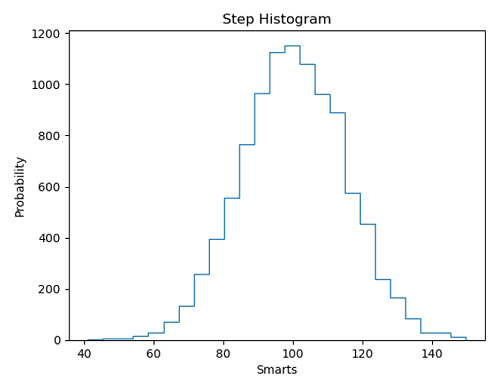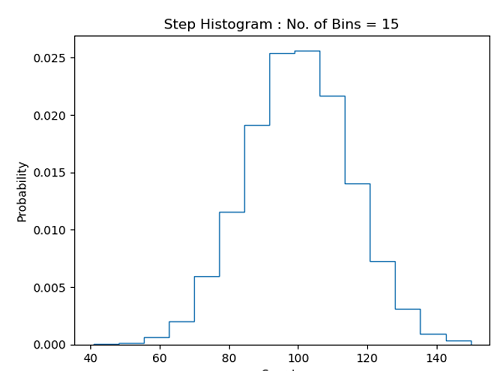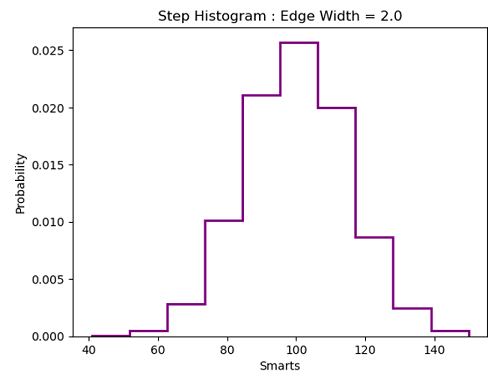# Python | Step Histogram Plot

Step Histogram Plot in Python. Here, we are going to learn about the step histogram plot and its Python implementation.
Submitted by Anuj Singh, on July 19, 2020

A histogram is a graphical technique or a type of data representation using bars of different heights such that each bar group's numbers into ranges (bins or buckets). Taller the bar higher the data falls in that bin. A Histogram is one of the most used techniques in data visualization and therefore, matplotlib has provided a function matplotlib.pyplot.hist() for plotting histograms. Step histogram is a type of histogram in which Bars are not filled with color, instead only the edge is the representation of Histogram. It is in a form of steps of stairs and therefore it is known as step histogram.

The following example illustrates the implementation and use of the Step Histogram Plot.## Python code for step histogram plot

```import matplotlib.pyplot as plt
import numpy as np

# random data generation
mu, sigma = 100, 15
x = mu + sigma * np.random.randn(10000)

# Histogram of the Data
plt.figure()
plt.hist(x, 25, histtype='step')
plt.xlabel('Smarts')
plt.ylabel('Probability')
plt.title('Step Histogram')
plt.show()

plt.figure()
plt.hist(x, 15, density=1, histtype='step')
plt.xlabel('Smarts')
plt.ylabel('Probability')
plt.title('Step Histogram : No. of Bins = 15')
plt.show()

plt.figure()
plt.hist(x, 10, density=1, edgecolor='purple', linewidth=2.0, histtype='step')
plt.xlabel('Smarts')
plt.ylabel('Probability')
plt.title('Step Histogram : Edge Width = 2.0')
plt.show()
```

Output:

```Output is as figure
```